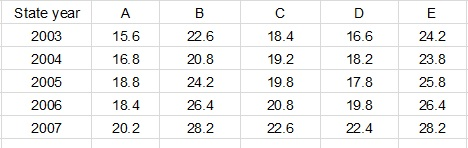## SBI PO Quant Test 13

Instructions

Study the following table carefully to answer these question
Population (in lakhs) of five states over the year
tableQuestion 1

What is the average population of state B in lakhs for all the years together ?

Question 2

What is the ratio of the population of state A in 2003 to the population of state E in 2007 ?

Question 3

The population of State E in 2004 is approximately what percent of the population of state D in 2006 ?

Question 4

What is the total population of all the states together in 2005 ?

Question 5

Population of state E in 2007 is what per cent of the total population of all the states together in that year ?(rounded off to nearest integer)